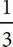# SAT Math Multiple Choice Question 185: Answer and Explanation

### Test Information

Question: 185

5. 2s -t = 10
5s = t + 12 - s

Which of the following is a true statement about the system of equations above?

• A. There are infinitely many solutions to the system of equations.
• B. When the system is solved for s, the result is 5.
• C. When the system is solved for t, the result is 6.
• D. There are no solutions to the system of equations.